# Series (mathematics)

In mathematics, a series is the cumulative sum of a given sequence of terms. Typically, these terms are real or complex numbers, but much more generality is possible.

For example, given the sequence of the natural numbers 1, 2, 3, ..., the series is
1,
1 + 2,
1 + 2 + 3, ...
The above writing stresses the 'cumulative' nature of the series and is justified by the mathematical definition we introduce below, but more direct notation is typically used: 1 + 2 + 3 + ... .

Depending on the number of terms, the series may be finite or infinite. The former is relatively easy to deal with. The finite series is identified with the sum of all terms and — apart from the elementary algebra — there is no particular theory that applies. It turns out, however, that much care is required when manipulating infinite series. For example, some simple operations borrowed from the elementary algebra — such as a change of order of the terms — often lead to unexpected results. So it is sometimes tacitly understood, especially in analysis, that the term "series" refers to the infinite series. In what follows we adopt this convention and concentrate on the theory of the infinite case.

## Motivations and examples

Given a series, an obvious question arises. Does it make sense to talk about the sum of all terms? That is not always the case. Consider a simple example when the general term is constant and equal to 1, say. That is, the series reads as 1+1+1+1+... (without end). Clearly, the sum of all terms can not be finite. In mathematical language, the series diverges to infinity.  There is not much to say about such an object. If we want to build an interesting theory, that is to have some examples, operations and theorems, we need to deal with convergent series, that is series for which the sum of all terms is well-defined.

Actually, are there any? Maybe any series would diverge like this? Consider the following series of decreasing terms, where every term is half the previous term: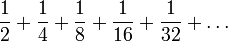$\frac{1}{2}+\frac{1}{4}+\frac{1}{8} +\frac{1}{16} +\frac{1}{32} + \ldots$

This is a special example of what is called geometric series. The sum is finite! Instead of a rigorous proof, we present a picture (see fig.1) which gives a geometric interpretation. Each term is represented by a rectangle of the corresponding area. At any moment (given any number of rectangular "chips on the table"), the next rectangle covers exactly one half of the remaining space. Thus, the chips will never cover more than the unit square. In other words, the sum increases when more terms are added, but it does not go to the infinity, it never exceeds 1. The series converges.

One may tend to think that any decreasing sequence of terms would eventually lead to a convergent series. This, however, is not the case. Consider for example the series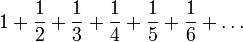$1+\frac{1}{2}+\frac{1}{3}+\frac{1}{4}+\frac{1}{5}+\frac{1}{6}+\ldots$

To see that it diverges to infinity, let us make the following groups of terms (we may and do forget the one at the beginning):

1/2,
1/3+1/4,
1/5+1/6+1/7+1/8,
1/9+1/10+1/11+1/12+1/13+1/14+1/15+1/16,

etc. We will show that sum of each line is at least 1/2. Notice that each group ends by a term of the form 1 / 2n, the smallest one in the group. Note also that group that ends by 1 / 4 has two members, the one that ends by 1 / 8 has four members and so on. Generally, a group that ends by 1 / 2n has 2n − 1 terms, each of which is greater than the smallest one at the end. So pick a group and replace each term by the smallest one. Then the new sum is easy to calculate:

2n − 1 members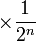$\times \frac{1}{2^n}$ (this is the smallest term) = 1 / 2

Obviously, the original sum is at least as big as this and our claim follows: the sum of each group is at least one half. And since there are infinitely many of such groups, the sum of all terms is not finite.

These examples show that we need criteria to decide whether a given series is convergent. Before such tools can be developed we need some mathematical notation.

## Formal definition

Given a sequence$a_1, a_2,\dots$ of numbers (or other mathematical objects that can be added), let$S_n=a_1+a_2+\cdots+a_n,\qquad n\in\mathbb{N}.$

Then, the series is defined as the sequence$\{S_n\}_{n=1}^\infty$ and denoted by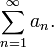$\sum_{n=1}^\infty a_n.$ For a single n, the sum Sn is called the partial sum of the series.

If the sequence (Sn) has a finite limit, the series is said to be convergent. In this case we define the sum of the series as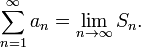$\sum_{n=1}^\infty a_n = \lim_{n\to\infty}S_n.$

Note that the sum (i.e. the numeric value of the above limit) and the series (i.e. the sequence Sn) are usually denoted by the same symbol. If the above limit does not exist - or is infinite - the series is said to be divergent.

## Series with positive terms

The simplest to investigate are the series with positive terms$(a_n\ge 0).$ In this case, the cumulative sum can only increase. The only question is whether the growth has a limit. This simplifies the analysis and results in a number of basic criteria. Notice that it is not positivity that really matters. If a given series has only negative terms, a "symmetric" argument can always be applied. So the only thing we really need is the constant sign. However, for the sake of clarity, in this section we assume that the terms are simply positive.

### Comparisons

There is a "family" of relative tests. The nature of a given series is inferred from what we know about another series, possibly simpler one.

If for two series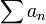$\sum a_n$ and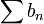$\sum b_n$ we have$a_n \le b_n$ and if the series$\sum b_n$ is known to be convergent, then the other one,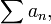$\sum a_n,$ is convergent as well. In other words a series "smaller" than a convergent one is always convergent. This is not difficult to justify. When we consider series of positive terms, the cumulative sum may only increase. For convergent series the growth is not unlimited -- there is an upper bound. Accordingly, the growth of the "smaller" series is limited by the same bound.

The above argument works the other way round too. If for the two series we have$a_n \ge b_n$ and the series$\sum b_n$ is known to be divergent, then the other one,$\sum a_n,$ diverges as well. In other words a series "bigger" than a divergent one is divergent too.

A very useful version of comparison test may be expressed as follows. If for two series$\sum a_n$ and$\sum b_n$ it can be established that$\lim_{n\to \infty}\frac{a_n}{b_n} = c$

with a finite strictly positive constant$c\in(0,\infty)$, then both series are of the same nature. In other words, if one series is known to converge, the other converges as well; if one diverges, so does the other.

Comparison with integral. This can be done if the sequence of general terms is decreasing, that is an > an + 1. More precisely, suppose that we have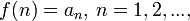$f(n)=a_n,\, n=1,2,...,$ for a certain decreasing function f defined on$[1,\infty)$. If the integral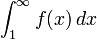$\int_1^\infty f(x) \, dx$

converges, so does the series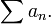$\sum a_n.$

This equivalence is quite important since it allows to establish convergence of a given series using basic methods of calculus. Prominent examples include the Riemann series, that is the series with general term an = 1 / np with a constant$p\in (0,\infty).$ If we take the function f(x) = 1 / xp then we get$\int_1^\infty f(x) \, dx = [ \ln x ]_1^\infty$ if p = 1 and$\int_1^\infty f(x) \, dx = \left[ \frac{x^{-p+1}}{-p+1} \right]_1^\infty$ otherwise.

The limit at infinity exists if and only if p > 1. It follows that the series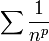$\sum \frac{1}{n^p}$

converges if and only if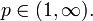$p\in (1,\infty).$

### Absolute tests

There are also tests that allow to determine the nature of a given single series. Two most popular are listed below.

In its simplest form it involves computation of the following limit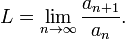$L=\lim_{n\to \infty} \frac{a_{n+1}}{a_n}.$

If L is strictly greater than 1, then the series diverges. If L < 1 then the series is convergent. The case L = 1 gives no answer. Indeed, take for example an = 1 / n. A short computation gives L = 1. Notice that we showed that this series is divergent. On the other hand, if an = 1 / n2, the series was shown to converge. Still, if we compute L from the above formula, we get L = 1.

In its popular form it is based on the computation of the following number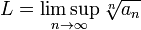$L=\limsup_{n\to\infty} \sqrt[n]{a_n}$

(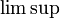$\limsup$ here refers to the upper limit of the sequence). Similarly as before, L > 1 implies divergence, L < 1 means that the series is convergent. The case L= 1 does not allow to decide.

Which one of the two above tests is more appropriate depends on the form of the general term. However, not only computational convenience is in question. In some cases only the root test can be applied. Consider for example the series 1 + 1 + 1/2 + 1/2 + 1/4 + 1/4 + 1/8 + 1/8 + 1/16 + 1/16 +.... Formally, the limit of the ratio an + 1 / an does not exist (the upper limit is equal to 1, the lower limit is 1/2). Nonetheless the root test gives$L = 1/\sqrt{2}$ and this determines that the series converges.

In general, the root test is more universal in the following sense: if the limit L of the ratios an + 1 / an exists, then the limit of roots$\sqrt[n]{a_n}$ exists as well and both are equal. On the other hand, if the ratio test fails to give an answer due to L = 1, then there is no hope that the root test allows to decide.

## Series with arbitrary terms

In general, a given series may contain terms of different sign. This may lead to very delicate situations. Consider a simple example, called the alternating harmonic series$1-\frac{1}{2}+\frac{1}{3}-\frac{1}{4}+\ldots.$

Denote the general term by an = ( − 1)n − 1 / n. Imagine that each term represents a small step along a line. Considering the sign, we swing back and forth. And it is easy to see that due to shortening the step we will approach a certain point, just like a damped pendulum -- see Fig. 2. This informally shows that$\sum a_n$ converges. Furthermore, we know the actual sum of this series, it equals to ln2 (this needs some actual computations, though). Now we reorganise the terms and consider$1-\frac{1}{2}-\frac{1}{4}+\frac{1}{3}-\frac{1}{6}-\frac{1}{8}+\ldots..$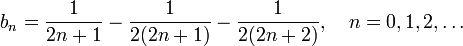$b_n = \frac{1}{2n+1}-\frac{1}{2(2n+1)}-\frac{1}{2(2n+2)},\quad n=0,1,2,\ldots$

Clearly, this series contains the same terms as$\sum a_n$ just in different order. Furthermore, simplifying the expression for bn gives$b_n=\frac{1}{2(2n+1)} - \frac{1}{2(2n+2)} = \frac{1}{2}\left( \frac{1}{2n+1} - \frac{1}{2n+2}\right)$

so that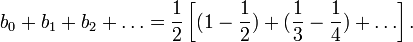$b_0+b_1+b_2+\ldots = \frac{1}{2} \left[ (1-\frac{1}{2}) + (\frac{1}{3}-\frac{1}{4}) + \ldots \right].$

It follows that the total sum is$1/2\cdot \ln 2$. And we changed only the order...

Even a more general result can be shown: for any given number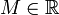$M\in\mathbb{R}$ we can find a rearrangement of terms in our series$\sum a_n$ to get the total sum of M.

To assure a more regular behaviour the notion of absolute convergence is introduced. We say that a series$\sum a_n$ is absolutely convergent if the series$\sum |a_n|$ converges. The absolute convergence is stronger that the simple convergence introduced at the beginning. This means that any absolutely convergent series is convergent in the former sense. Consequently, given a series with arbitrary terms an one may apply any of the above mentioned basic criteria to | an | . If the result is positive ("convergence" detected) it means that the series is absolutely convergent -- so it converges in the sense of the first definition as well. Furthermore, the absolutely convergent series do not change the sum on reordering terms.

However, as we showed there are interesting series that are convergent but not absolutely convergent. Typical examples include alternating series, that is series whose general term each time changes the sign: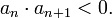$a_n\cdot a_{n+1} < 0.$ For these we have a criterion.

• If | an | form a decreasing sequence and the sign of an is alternating then the series$\sum a_n$ is convergent.

With this useful tool we immediately see that our alternating harmonic series converges. This is not very surprising -- since an actual proof of the criterion is just mathematicaly rigorous transcription of what can be seen on the Fig.2.

Notice that the relative tests from the previous section do not apply for alternating or arbitrary series (unless one investigates the modulus of the general term and the absolute convergence question). Consider the following examples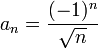$a_n=\frac{(-1)^n}{\sqrt{n}}$$b_n=\frac{(-1)^n}{(-1)^n+\sqrt{n}}$

The general terms are obviously equivalent (the ratio an / bn converges to 1). The first series converges in virtue of our last criterion while the second one may be shown to diverge.

We can invert the question we dealt with so far ("what can guarantee the convegence") and ask what follows from the convergence of a series. What all convergent series have in common? Here is an answer.

• If$\sum a_n$ is convergent then$\lim_{n\to\infty} a_n =0.$

That is, the general numbers, taken as a sequence, converge to 0. For example, the series with the general term$a_n=(-1)^n \frac{2n+1}{4n-5}$ or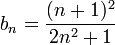$b_n=\frac{(n+1)^2}{2n^2+1}$

can not be convergent.

## Series of functions

On one hand, the sum of a series$f_1+f_2+\dots$ of functions fn is defined in the same way as above, — as the limit of the sequence of functions$S_n=f_1+\dots+f_n$, the partial sums of the series. On the other hand, convergence of functions differs strikingly from convergence of numbers. There is only one widely used convergence mode for numbers; in contrast, there are several widely used convergence modes for functions.

It is convenient to reduce the general convergence,$S_n \to S$, to the special case,$R_n \to 0$, letting Rn = SnS.

For example, the sequence of functions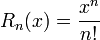$R_n(x) = \frac{x^n}{n!}$

converges to 0 pointwise but not uniformly. It means that, given$\epsilon > 0$ and x, there exists N such that$|R_n(x)| < \epsilon$ for all n > N. However,N depends on x, and no N can serve all x. In other words: Rn(x) converges to 0 for every x (which means, x not depending on n), but Rn(xn) need not converge to 0 for arbitrary sequences (xn). For instance, xn = n leads to$R_n(x_n) = n^n/n! \to \infty$.

Every example of the sequence of functions Rn is relevant to the general theory of functional series, since it corresponds to some example of the series of functions$f_1+f_2+\dots$, namely, fn = RnRn − 1.

For a sequence of continuous functions on [0,1], still, uniform convergence does not follow from pointwise convergence. For example, functions

Rn(x) = xnx2n for 0 ≤ x ≤ 1.

Choosing xn such that (xn)n = 0.5 one gets Rn(xn) = 0.25 in spite of pointwise convergence to 0. Here is a similar example for periodic functions on the whole line:

Rn(x) = sinnx − sin3nx.

The uniform convergence of Rn to 0 can be written in the form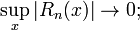$\sup_x |R_n(x)| \to 0;$

usual convergence of some numeric characteristics of these functions. Many other modes of convergence are also of this form. Some examples:$\int |R_n(x)| \, dx \to 0;$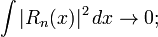$\int |R_n(x)|^2 \, dx \to 0;$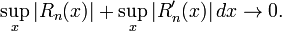$\sup_x |R_n(x)| + \sup_x |R'_n(x)| \, dx \to 0.$

Most important are two classes of series of functions: power (especially, Taylor) series whose terms are power functions cnxn and trigonometric (especially, Fourier) series whose terms are trigonometric functions ancosnx + bnsinnx; the latter being the real part of the complex number cneinx for cn = anibn, the series with terms cneinx is also treated as Fourier series.

These two classes are unrelated when x is real, but related via complex numbers: if$x = e^{i\phi}$ then$c_n x^n = c_n e^{in\phi}$.

Taylor and Fourier series behave quite differently. A Taylor series converges uniformly, together with all derivatives, on [ − a,a] whenever a is less than the radius of convergence, and its sum is a smooth (more exactly, analytic, therefore, continuous and infinitely differentiable) function. The behavior of a Fourier series depends on the behavior of its sum. If the sum is smooth enough, the series converges uniformly, together with some derivatives. If the sum is only continuous, the series need not converge uniformly, nor even pointwise. If the sum is square integrable (maybe quite discontinuous), the series converges in the sense that$\int |R_n(x)|^2 \, dx \to 0$. Some distributions (generalized functions) also may be developed into Fourier series, in which case convergence is much weaker.

The different behavior of power and trigonometric series corresponds on the complex plane to the different behavior of a function, analytic on a disk, inside the disk and on its boundary. Inside the disk the function is always smooth; on the boundary it can be quite a bad function, and even something more general than a function.

## Notes and references

1. Adjective divergent series is used as well
2. A simple calculation shows that the sum is actually equal to one. More details in geometric series article.
3. It is often called harmonic series
4. Other popular (equivalent) definition describes the series as a formal (ordered) list of terms combined by the addition operator
5. This is called Riemann series theorem.
6. To verify this one may consider the difference of the two general terms.Some content on this page may previously have appeared on Citizendium.